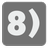Friendly Number
Elementary+
RU English FRLong numbers can be made to look nicer, so let’s write some code to do just that.

You should write a function for converting a number to string using several rules. First of all, you will need to cut the number with a given base ( base argument; default 1000). The value is a float number with decimal after the point ( decimals argument; default 0). For the value, use the rounding towards zero rule (5.6⇒5, -5.6⇒-5) if the decimal = 0, otherwise use the standard rounding procedure. If the number of decimals is greater than the current number of digits after dot, trail value with zeroes. The number should be a value with letters designating the power. You will be given a list of power designations ( powers argument; default ['', 'k', 'M', 'G', 'T', 'P', 'E', 'Z', 'Y']). If you are given suffix ( suffix argument; default ‘’) , then you must append it. If you don’t have enough powers - stay at the maximum. And zero is always zero without powers, but with suffix.

Let's look at examples. It will be simpler.

• n=102
result: "102", the base is default 1000 and 102 is lower this base.
• n=10240
result: "10k", the base is default 1000 and rounding down.
• n=12341234, decimals=1
result: "12.3M", one digit after the dot.
• n=12000000, decimals=3
result: "12.000M", trailing zeros.
• n=12461, decimals=1
result: "12.5k", standard rounding.
• n=1024000000, base=1024, suffix='iB'
result: '976MiB', the different base and the suffix.
• n=-150, base=100, powers=['', 'd', 'D']
result: '-1d', the negative number and rounding towards zero.
• n=-155, base=100, decimals=1, powers=['', 'd', 'D']
result: '-1.6d', the negative number and standard rounding.
• n=255000000000, powers=['', 'k', 'M']
result: '255000M', there is not enough powers.

Input: A number as an integer. The keyword argument "base" as an integer, default 1000. The keyword argument "decimals" as an integer, default 0. The keyword argument "suffix" as a string, default ''. The keyword argument "powers" as a list of string, default ['', 'k', 'M', 'G', 'T', 'P', 'E', 'Z', 'Y'].

Output: The converted number as a string.

Example:

```friendly_number(102) == '102'
friendly_number(10240) == '10k'
friendly_number(12341234, decimals=1) == '12.3M'
friendly_number(12000000, decimals=3) == '12.000M'
friendly_number(12461, decimals=1) == '12.5k'
friendly_number(1024000000, base=1024, suffix='iB') == '976MiB'
```

How it is used: In the physics and IT we have a lot of various numbers. Sometimes we need to make them more simpler and easier to read. When you talk about gigabytes sometimes you don’t need to know the exact number bytes or kilobytes.

Precondition: 1 < base ≤ 10 32
-10 32 < number ≤ 10 32
0 ≤ decimals ≤ 15
0 < len(powers) ≤ 32

Settings
Code:
CheckiO Extensions

CheckiO Extensions allow you to use local files to solve missions. More info in a blog post.

In order to install CheckiO client you'll need installed Python (version at least 3.8)

Install CheckiO Client first:

`pip3 install checkio_client`

`checkio --domain=py config --key=`

Sync solutions into your local folder

`checkio sync`

(in beta testing) Launch local server so your browser can use it and sync solution between local file end extension on the fly. (doesn't work for safari)

`checkio serv -d`

Alternatevly, you can install Chrome extension or FF addon

`checkio install-plugin`
`checkio install-plugin --ff`
`checkio install-plugin --chromium`

Read more here about other functionality that the checkio client provides. Feel free to submit an issue in case of any difficulties.

Sync...